# How to Visualize Time Series Data: Tidy Forecasting in R

Written by Joon Im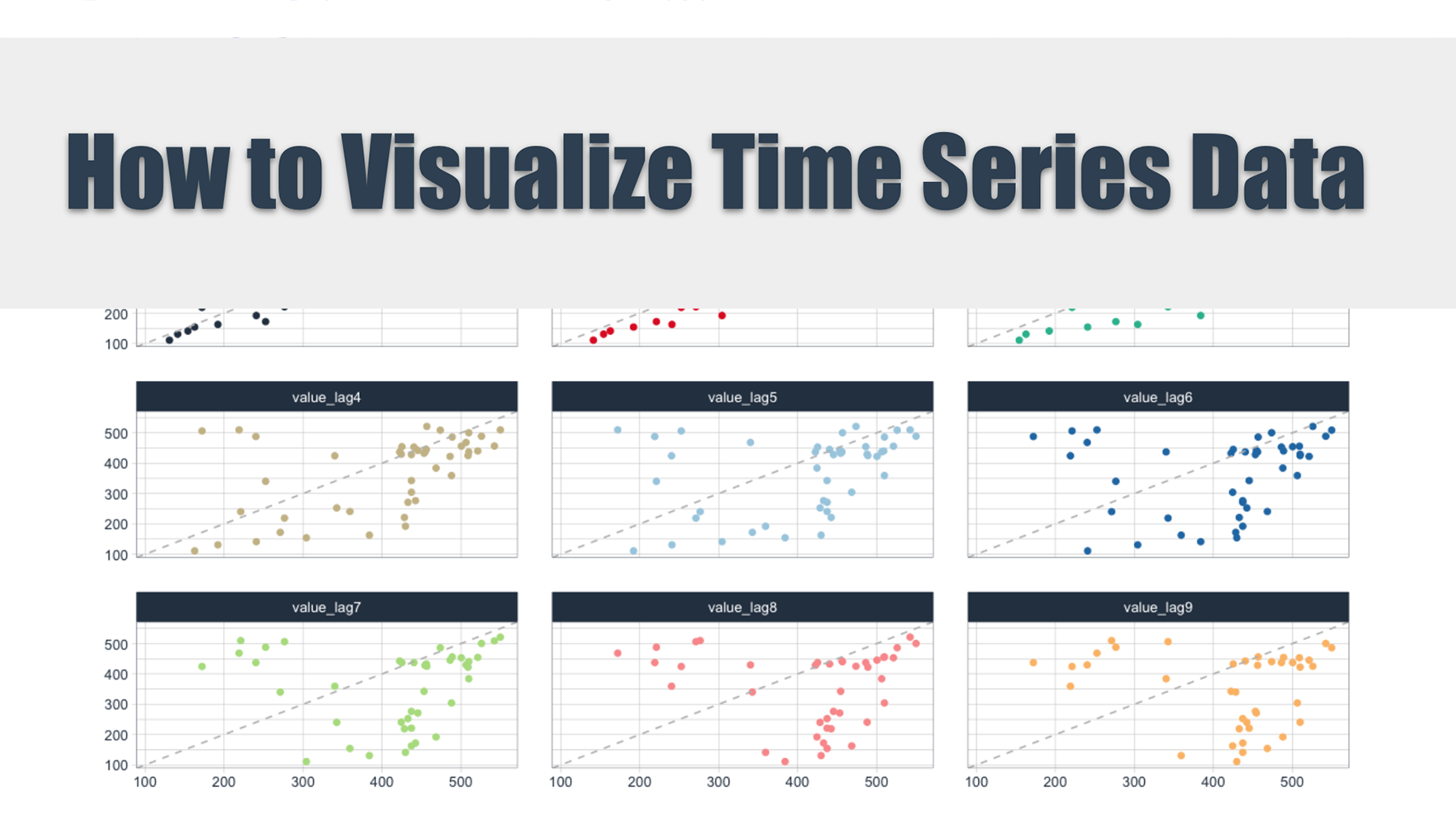# R Tutorials Update

Plot time series data using the fpp2, fpp3, and timetk forecasting frameworks.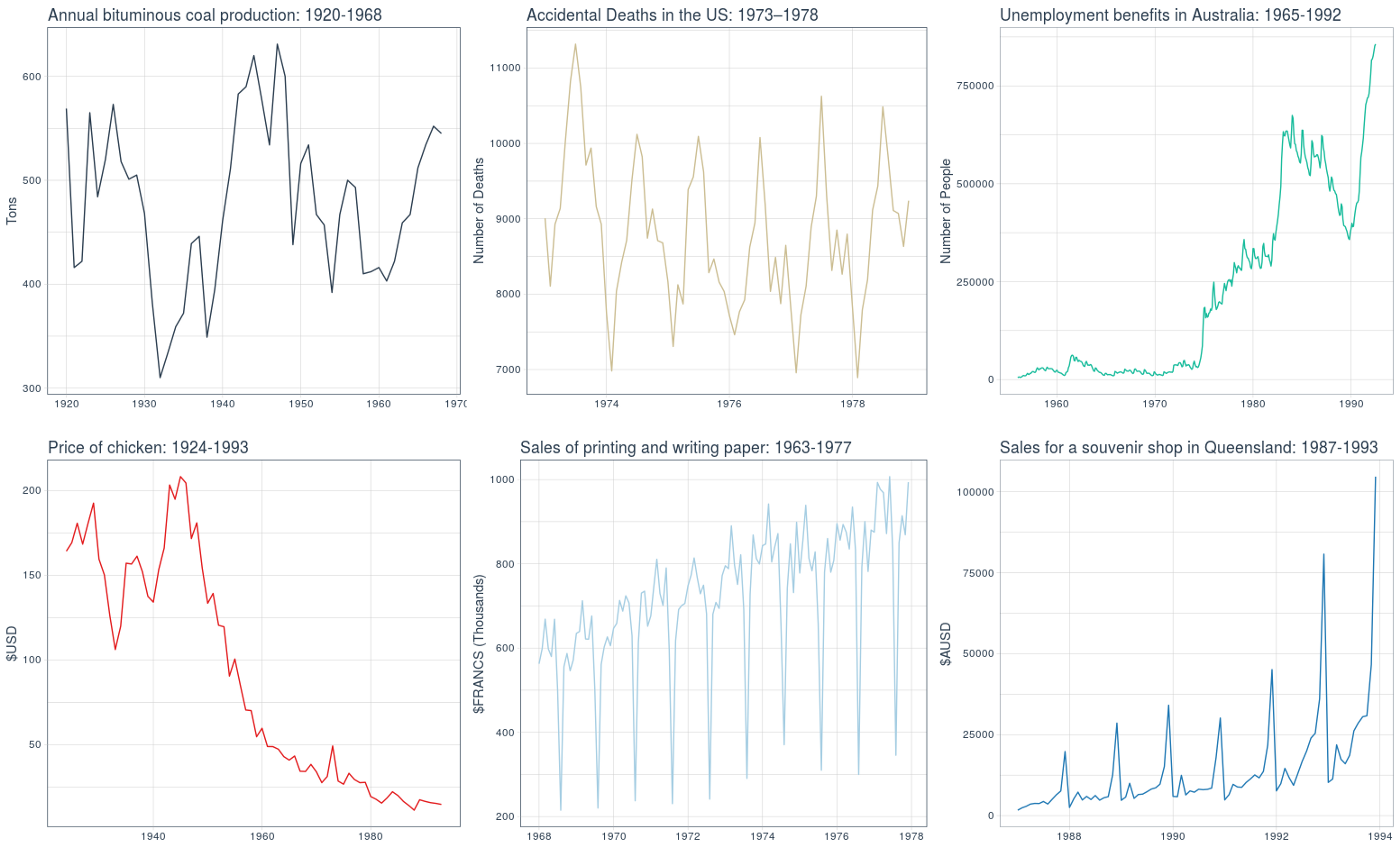# 1. Set Up

## 1.1. Introduction

There are a number of forecasting packages written in R to choose from, each with their own pros and cons.

For almost a decade, the forecast package has been a rock-solid framework for time series forecasting. However, within the last year or so an official updated version has been released named fable which now follows tidy methods as opposed to base R.

More recently, modeltime has been released and this also follows tidy methods. However, it is strictly used for modeling. For data manipulation and visualization, the timetk package will be used which is written by the same author as modeltime.

The following is a code comparison of various time series visualizations between these frameworks: fpp2, fpp3 and timetk.

A few things to keep in mind:

• Only the essential code has been provided
• Non-essential code such as plot titles and themes has been excluded
• All plots utilize the Business Science ggplot theme

# 2. TS vs tsibble

• The base ts object is used by forecast & fpp2
• The special tsibble object is used by fable & fpp3
• The standard tibble object is used by timetk & modeltime

## 2.1 Load Time Series Data

For the next few visualizations, we will utilize a dataset containing quarterly production values of certain commodities in Australia.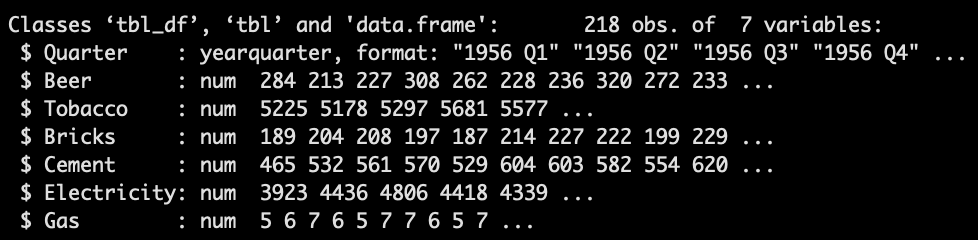Always check the class of your time series data.

## 2.2 fpp2 Method: From tibble to ts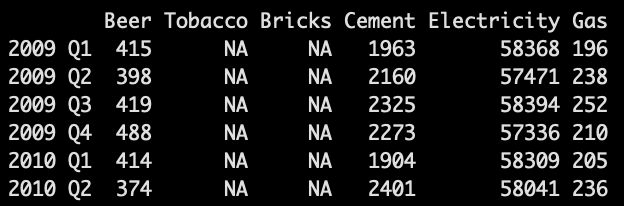## 2.3 fpp3 Method: From ts to tsibble

### 2.3.1 Pivot Wide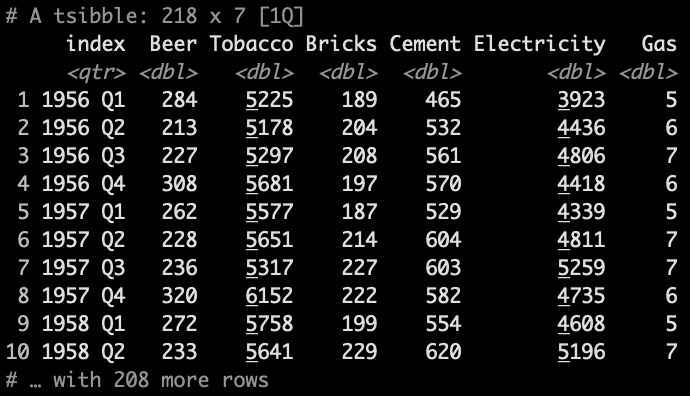### 2.3.2 Pivot Long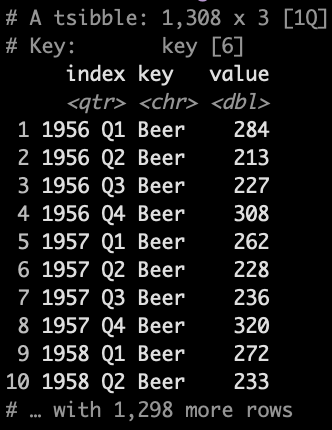## 2.4 timetk Method: From tsibble/ts to tibble

### 2.4.1 Pivot Wide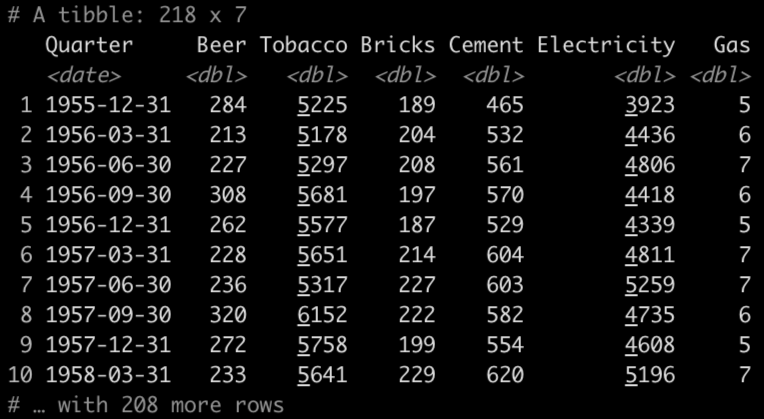Workaround for indexing issue with tsibble and R 4.0 and up.

### 2.4.2 Pivot Long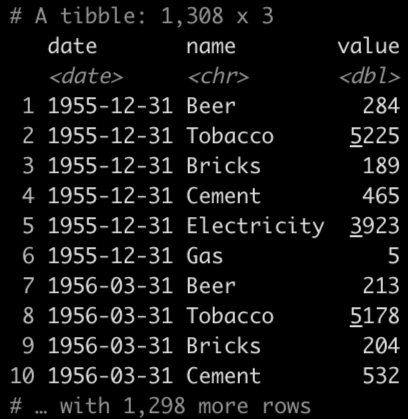# 3. Time Series Plots

When analyzing time series plots, look for the following patterns:

• Trend: A long-term increase or decrease in the data; a “changing direction”.

• Seasonality: A seasonal pattern of a fixed and known period. If the frequency is unchanging and associated with some aspect of the calendar, then the pattern is seasonal.

• Cycle: A rise and fall pattern not of a fixed frequency. If the fluctuations are not of a fixed frequency then they are cyclic.

• Seasonal vs Cyclic: Cyclic patterns are longer and more variable than seasonal patterns in general.

## 3.1 fpp2 Method: Plot Multiple Series On Same Axes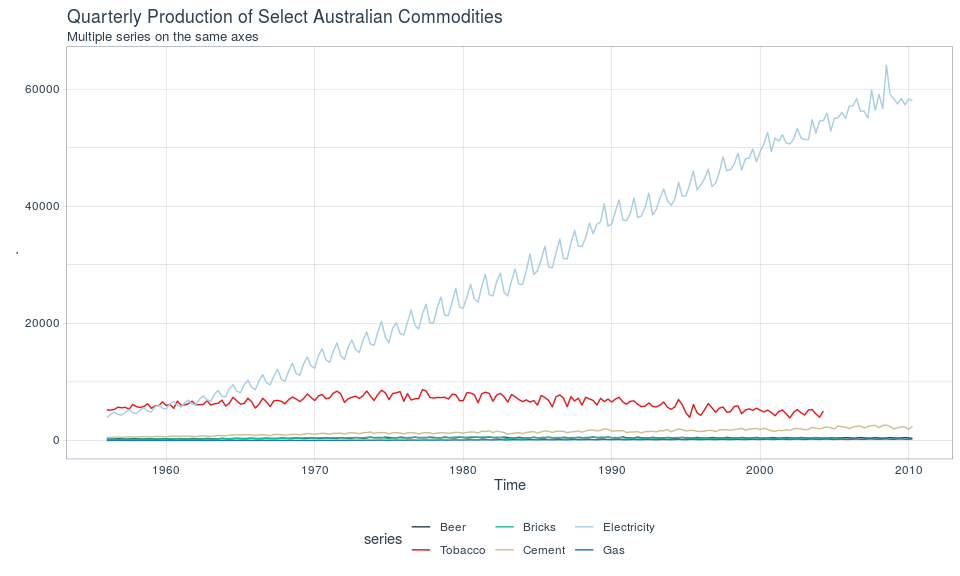## 3.2 fpp3 Method: Plot Multiple Series On Same Axes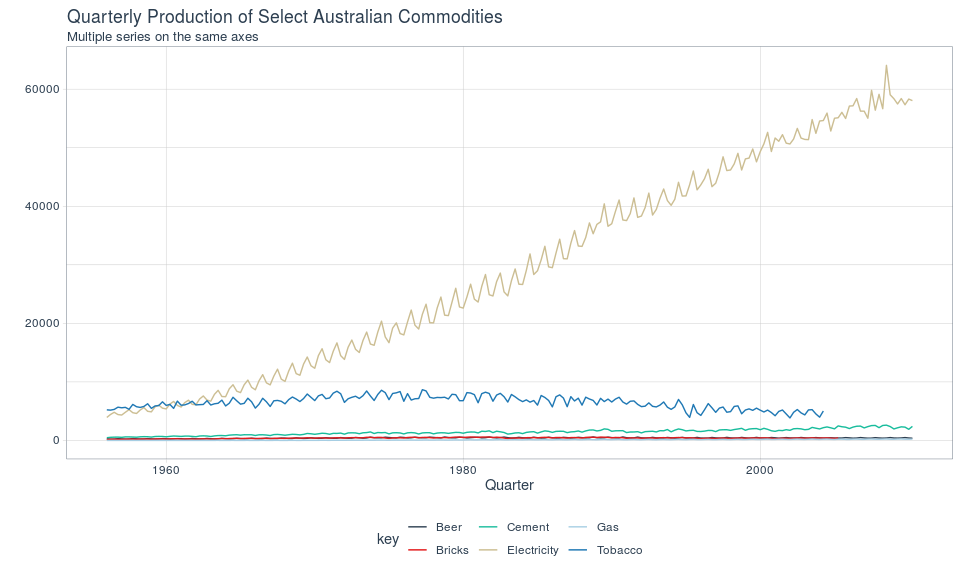## 3.3 ggplot Method: Plot Multiple Series On Same Axes

Note that plotting multiple plots on the same axes has not been implemented into timetk. Use ggplot.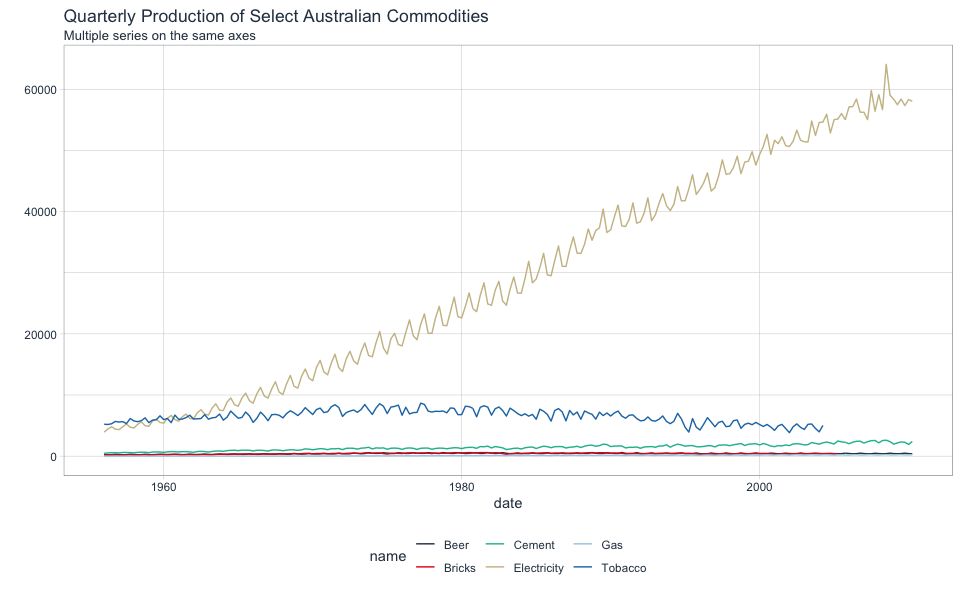## 3.4 fpp2 Method: Plot Multiple Series On Separate Axes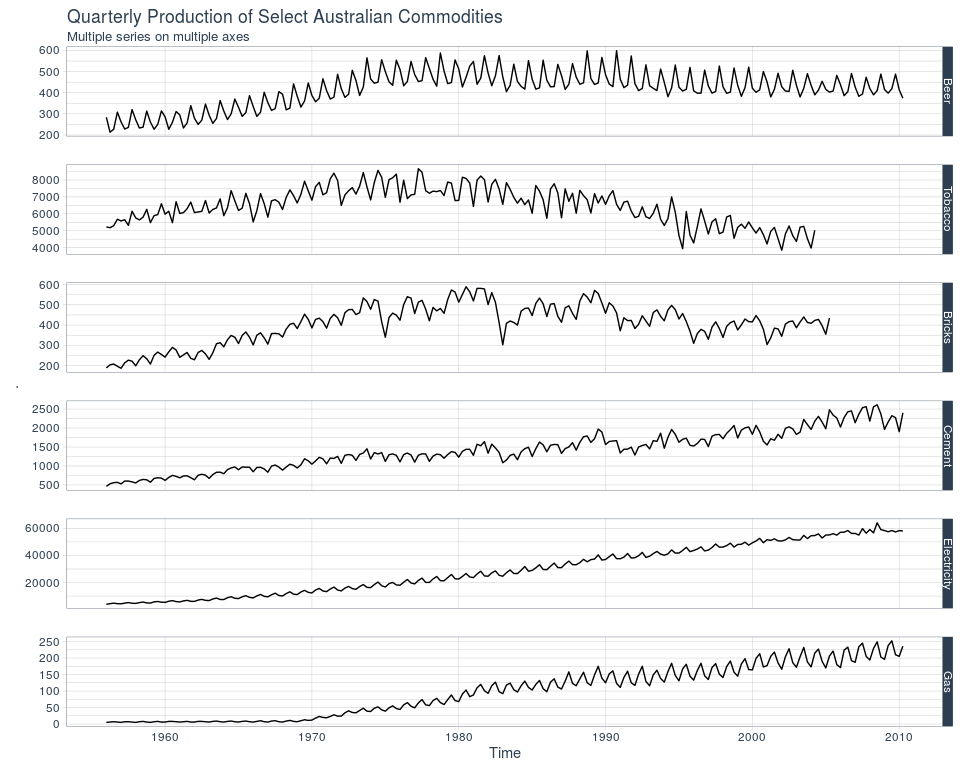Facetted plot with fpp2

## 3.5 fpp3 Method: Plot Multiple Series On Separate AxesFacetted plot with fpp3

## 3.6 timetk Method: Plot Multiple Series On Separate Axes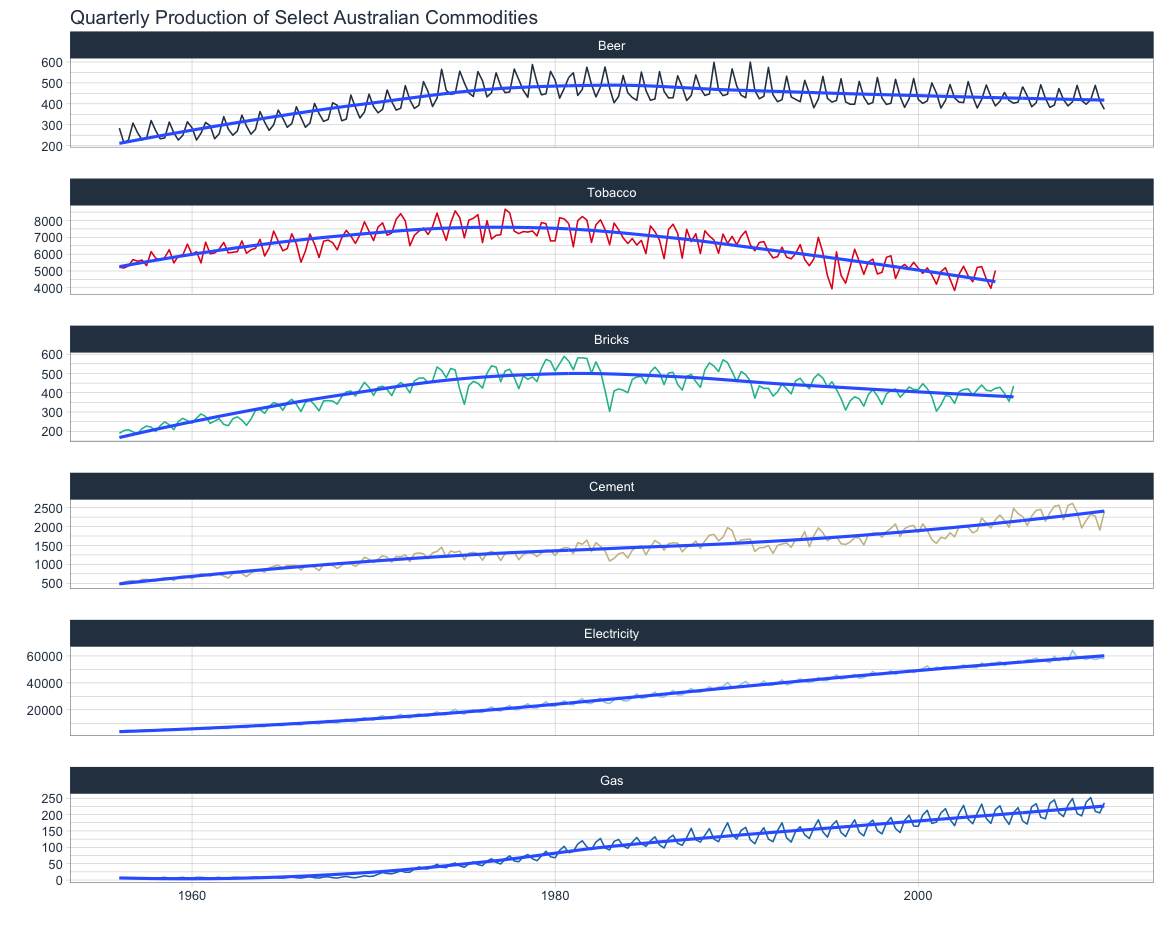Facetted plot with timetk

# 4. Seasonal Plots

Use seasonal plots for identifying time periods in which the patterns change.

## 4.1 fpp2 Method: Plot Individual Seasons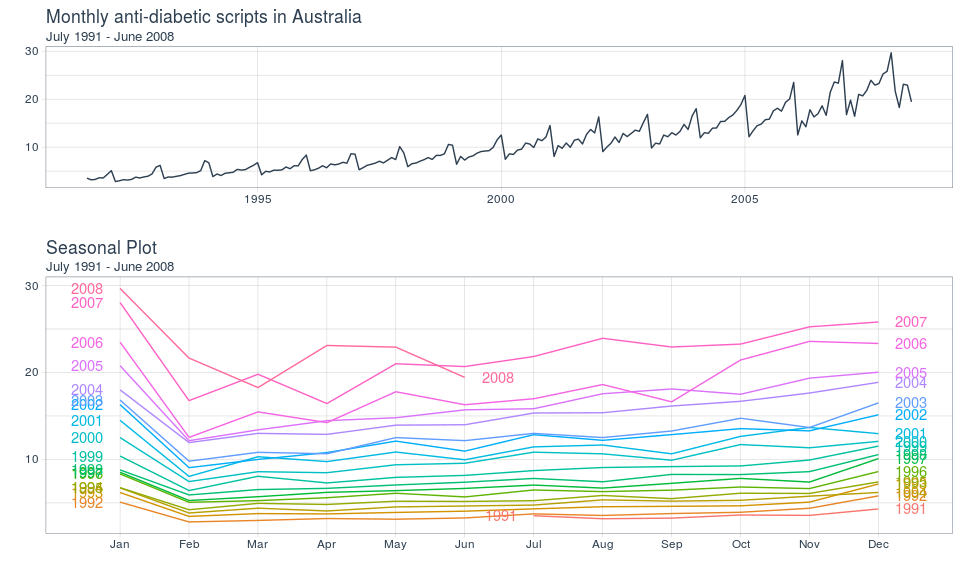Seasonal plot with fpp2

## 4.2 fpp3 Method: Plot Individual Seasons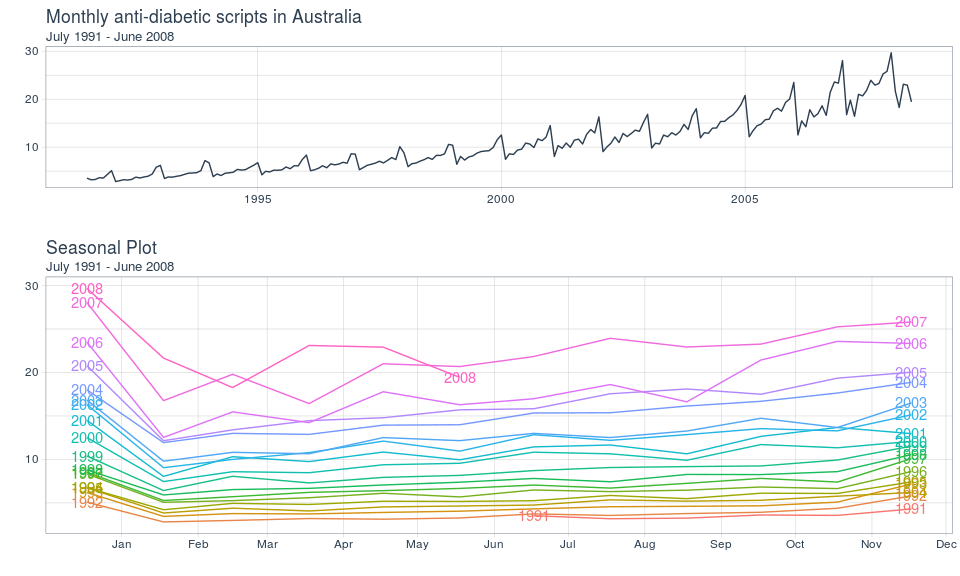Seasonal plot with fpp3

## 4.3 ggplot Method: Plot Individual Seasons

Note that seasonal plots have not been implemented into timetk. Use ggplot to write: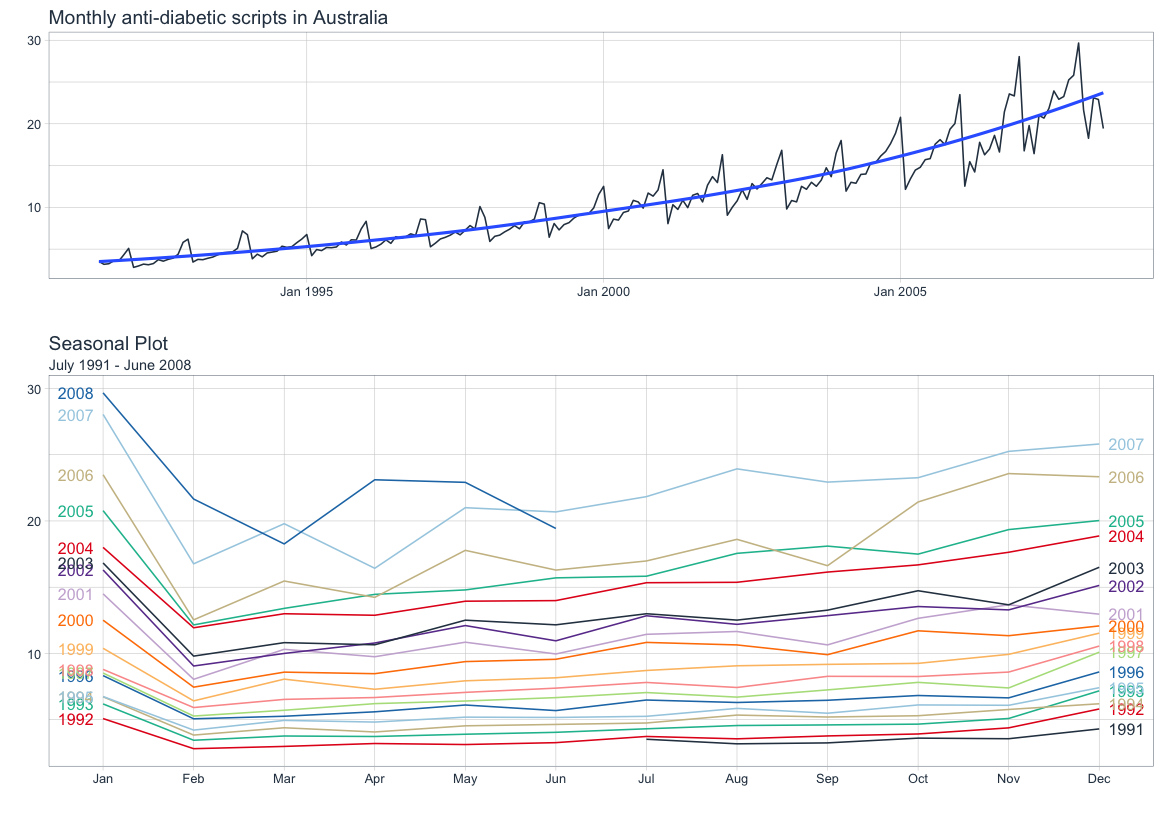Seasonal plot with ggplot

# 5. Subseries Plots

Use subseries plots to view seasonal changes over time.

## 5.1 fpp2 Method: Plot Subseries on Same Axes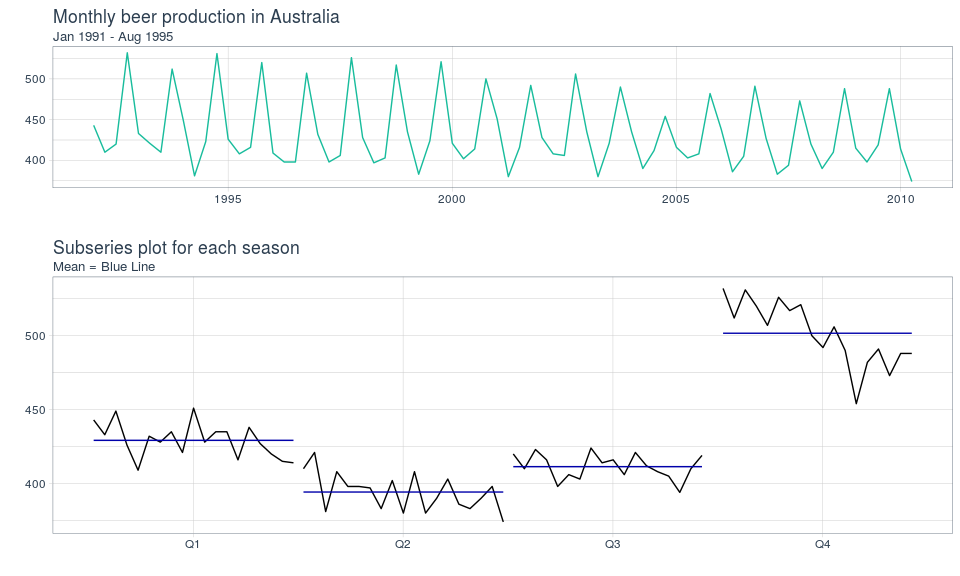Subseries plots on the same axes using fpp2

## 5.2 fpp3 Method: Plot Subseries on Separate Axes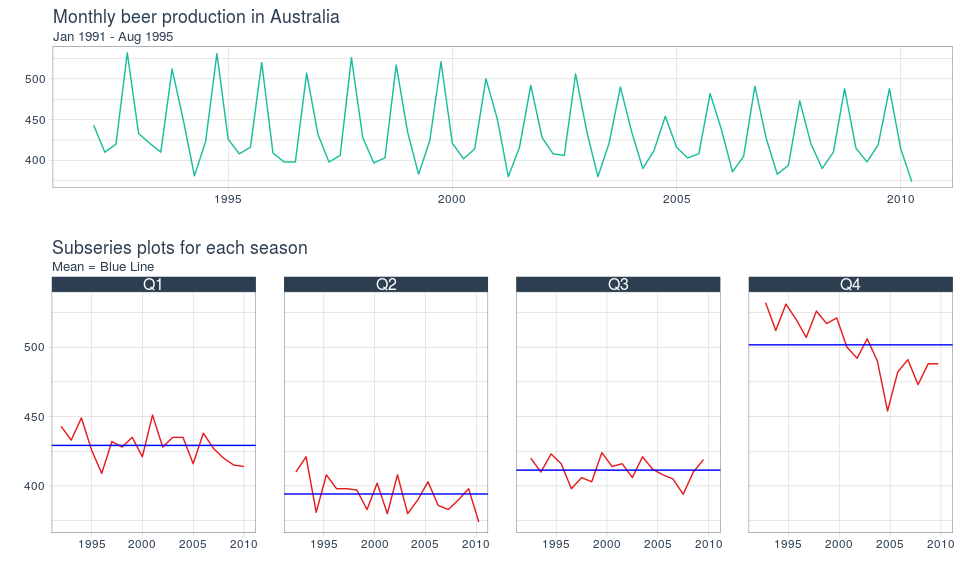Subseries plots on the same axes using fpp3

## 5.3 timetk Method: Plot Subseries on Separate Axes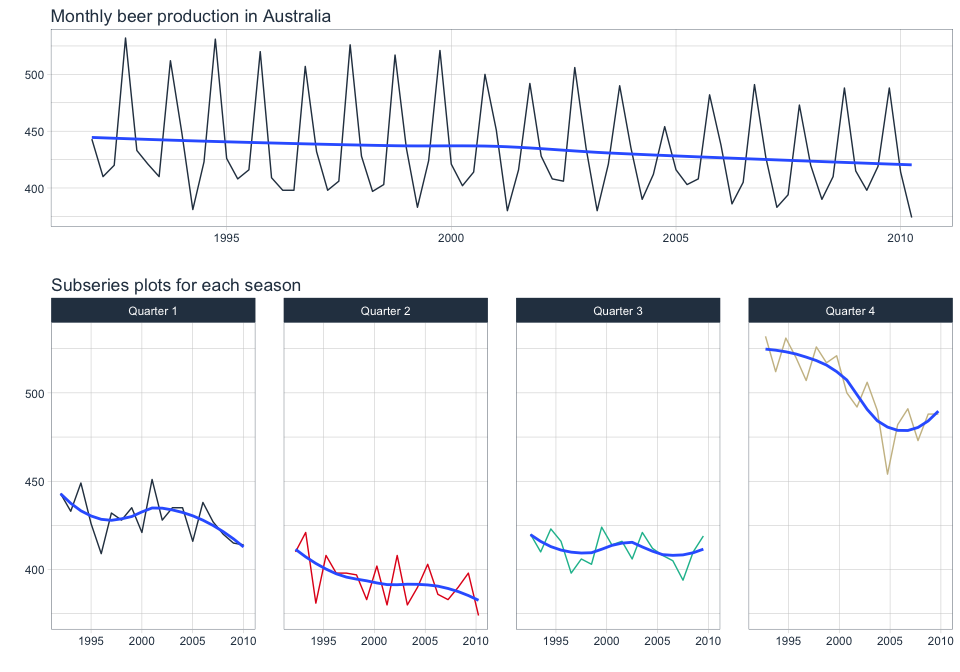Subseries plots on the same axes using timetk

# 6. Lag Plots

Use lag plots to check for randomness.

## 6.1 fpp2 Method: Plot Multiple Lags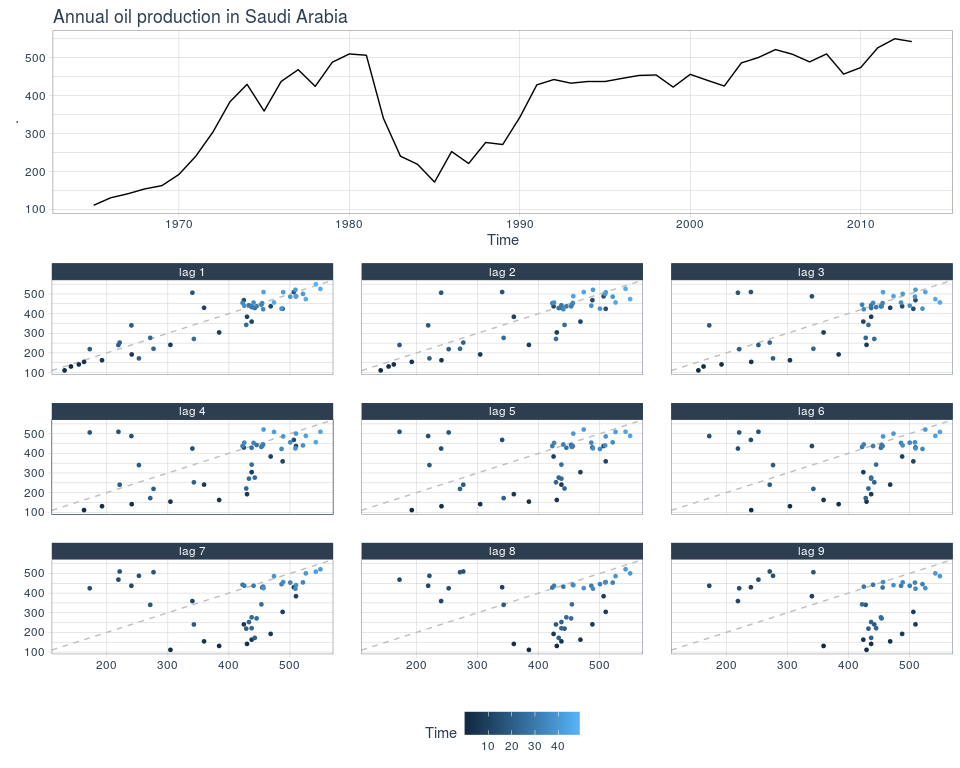Lag plots using fpp2

## 6.2 fpp3 Method: Plot Multiple Lags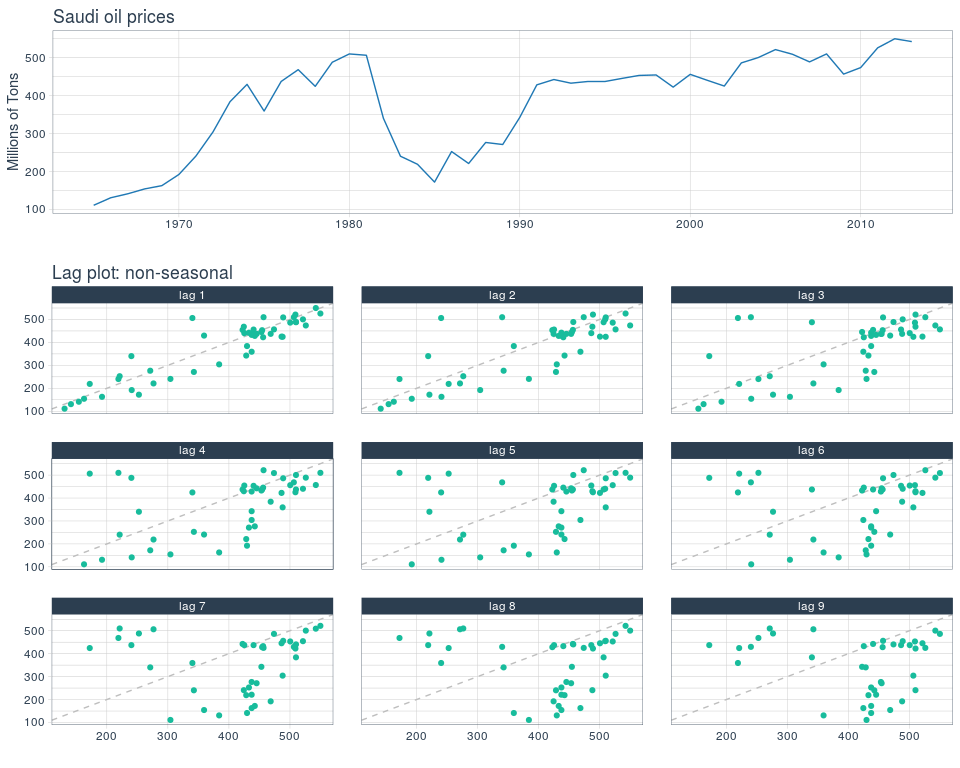Lag plots using fpp3

## 6.3 timetk Method (Hack?) : Plot Multiple Lags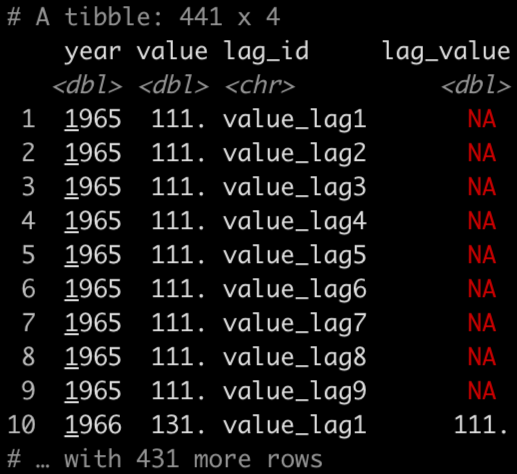Now you can plot value vs lag_value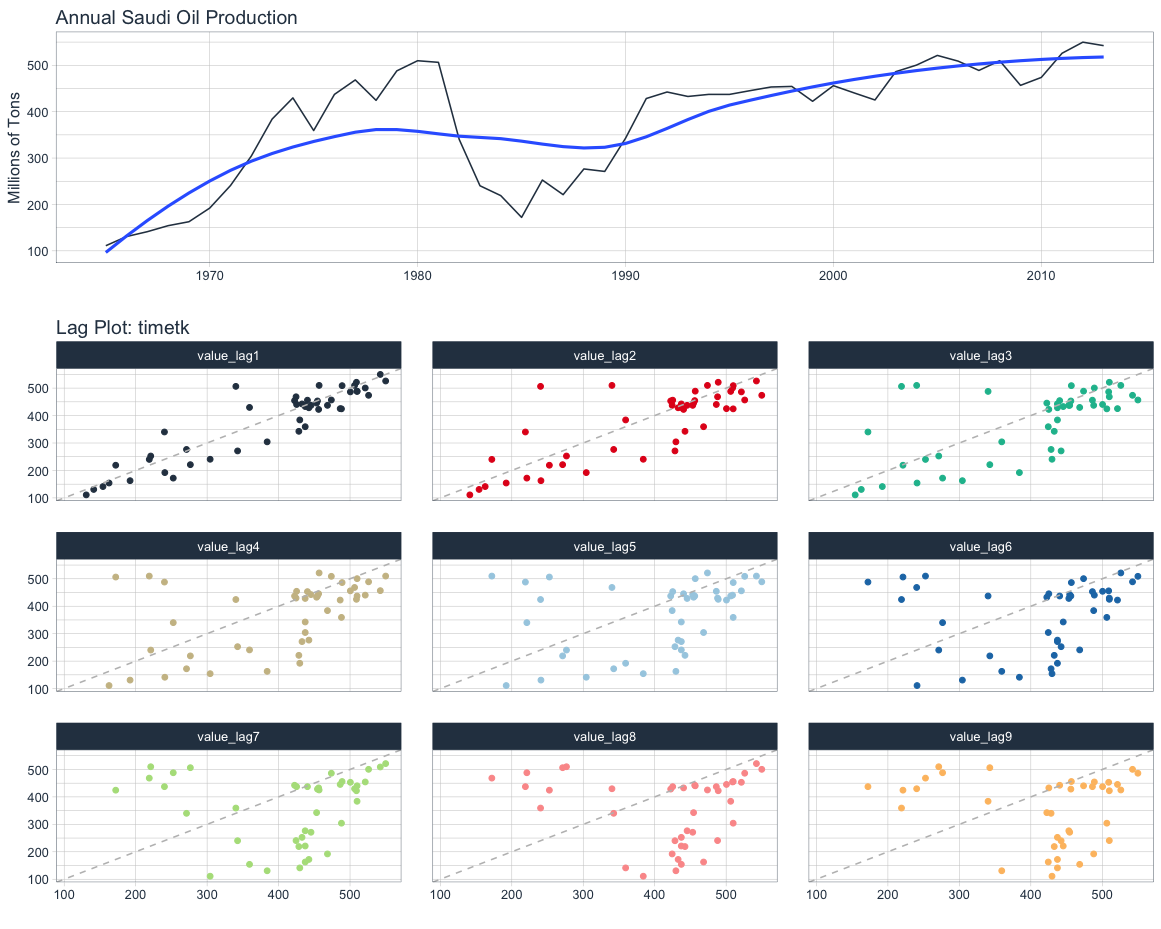Lag plots using timetk

# 7. Autocorrelation Function Plots

The autocorrelation function measures the linear relationship between lagged values of a time series. The partial autocorrelation function measures the linear relationship between the correlations of the residuals.

ACF

• Visualizes how much the most recent value of the series is correlated with past values of the series (lags)
• If the data has a trend, then the autocorrelations for small lags tend to be positive and large because observations nearby in time are also nearby in size
• If the data are seasonal, then the autocorrelations will be larger for seasonal lags at multiples of seasonal frequency than other lags

PACF

• Visualizes whether certain lags are good for modeling or not; useful for data with a seasonal pattern
• Removes dependence of lags on other lags by using the correlations of the residuals

## 7.1 fpp2 Method: Plot ACF + PACF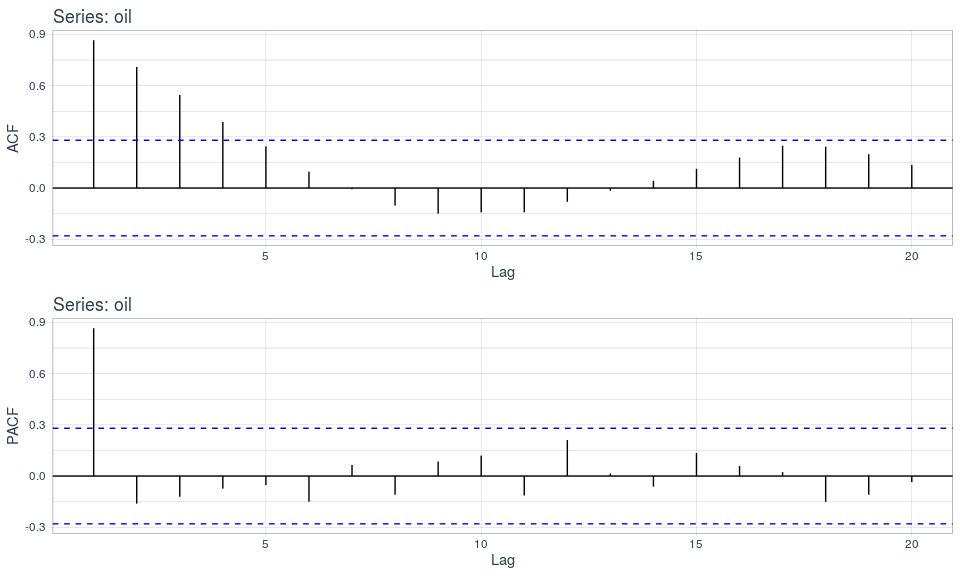Are autocorrelations large at seasonal lags? Are the most recent lags above the white noise threshold?

## 7.2 fpp3 Method: Plot ACF + PACF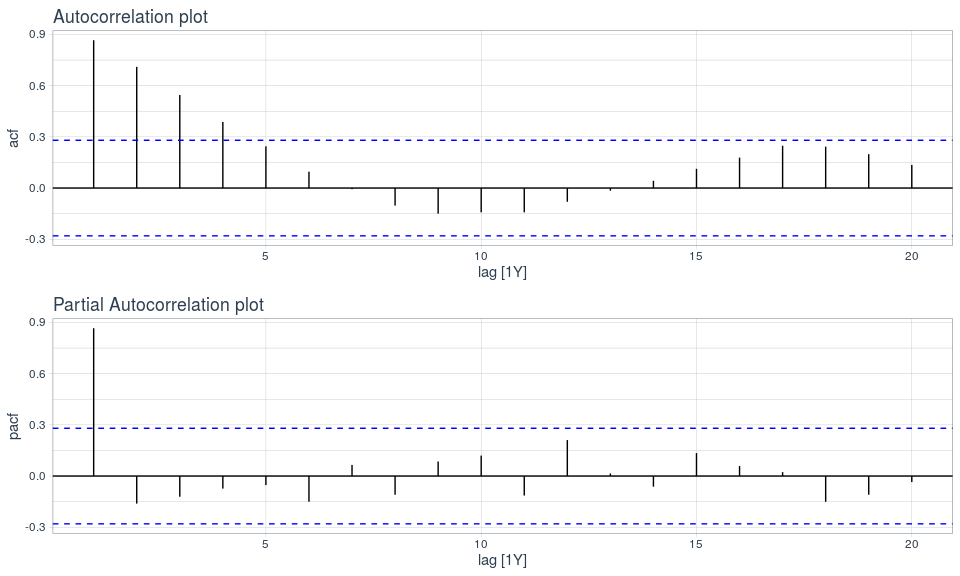The autocorrelations are not large at seasonal lags so this series is non-seasonal. The most recent lags show that there is a trend.

## 7.3 timetk Method: Plot ACF & PACF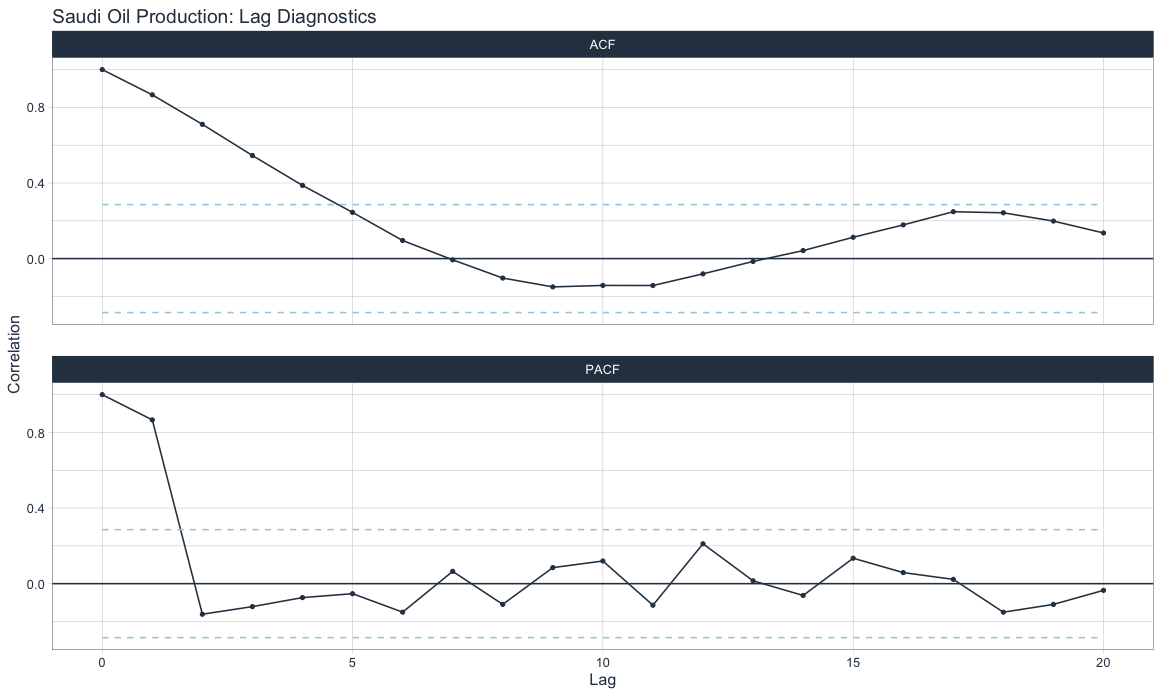ACF shows more recent lags are above the white noise significance bars denoting a trend. PACF shows that including lag 1 would be good for modeling purposes.

# 8. Summary

As with all things in life, there are good and bad sides to using any of these three forecasting frameworks for visualizing time series. All three have similar functionality as it relates to visualizations.

## 8.1 fpp2

• Code requires minimal parameters
• Uses basets format
• Uses ggplot for visualizations
• Mostly incompatible with tidyverse for data manipulation
• No longer maintained except for bug fixes

## 8.2 fpp3

• Code requires minimal parameters
• Uses proprietary tsibble format with special indexing tools
• Uses ggplot for visualizations
• Mostly compatible with tidyverse for data manipulation; tsibble may cause issues
• Currently maintained

## 8.3 timetk

• Code requires multiple parameters but provides more granularity
• Uses standard tibble format
• Uses ggplot and plotly for visualizations
• Fully compatible with tidyverse for data manipulation
• Currently maintained

Author: Joon Im
Joon is a data scientist with both R and Python with an emphasis on forecasting techniques - LinkedIn.